Tuesday, June 5, 2018

Preserving node & edge labels after optimizing node rotation in `cophylo`

A phytools user contacted me the other day about an issue in which her bootstrap values, stored as node labels in a Newick string, did not align with the correct edges when plotted using `edgelabels.cophylo` on a visualized `"cophylo"` object.

As it turns out, this had nothing to do with `cophylo` & everything to do with the fact that she wanted to plot node labels using `edgelabels`.

First, here is a demo showing that `cophylo` preserves the correct order of node labels.

A simulated tree with node labels:

``````library(phytools)
tree<-pbtree(n=26,tip.label=LETTERS)
tree\$node.label<-letters[1:25]
``````

Now I'll plot that tree labeling the nodes with their labels & node indices:

``````plotTree(tree)
nodelabels(tree\$node.label,1:tree\$Nnode+Ntip(tree))
``````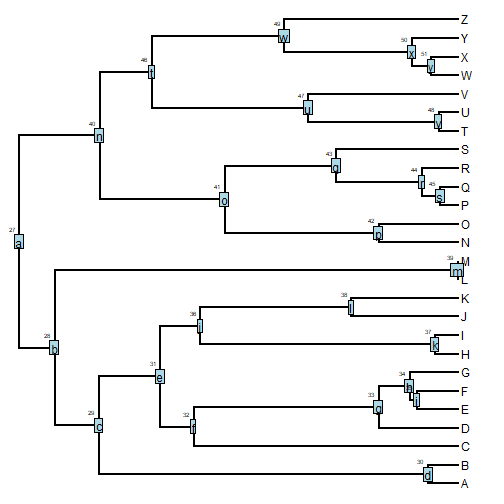Next, I'm going to randomly rotate nodes on this tree to produce two new trees as follows:

``````t1<-tree
for(i in 1:100) t1<-untangle(rotate(t1,sample(1:t1\$Nnode+Ntip(t1),1)),
t2<-tree
for(i in 1:100) t2<-untangle(rotate(t2,sample(1:t2\$Nnode+Ntip(t2),1)),
``````

Now I'm ready to make my `"cophylo"` object. First, without node rotation to maximize matching:

``````obj<-cophylo(t1,t2,rotate=FALSE)
plot(obj)
nodelabels.cophylo(obj\$trees[]\$node.label,1:obj\$trees[]\$Nnode+
Ntip(obj\$trees[]))
nodelabels.cophylo(obj\$trees[]\$node.label,1:obj\$trees[]\$Nnode+
Ntip(obj\$trees[]),which="right")
``````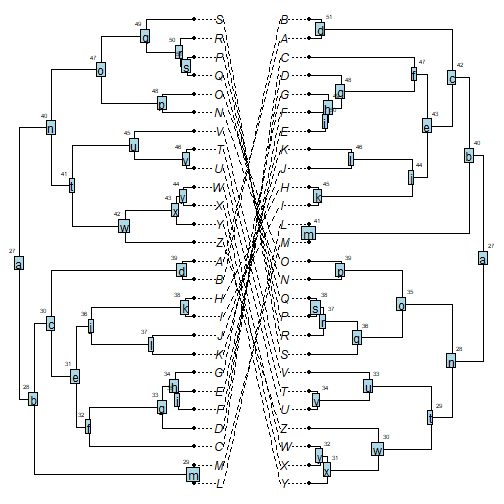We can see that the node indices (the little numbers that come from `tree\$edge` have changed, but the node labels are still all associated with the right clades.

Now we can do the same thing, but in which we permit node rotation to be optimized as follows:

``````obj<-cophylo(t1,t2,rotate=TRUE)
``````
``````## Rotating nodes to optimize matching...
## Done.
``````
``````plot(obj)
nodelabels.cophylo(obj\$trees[]\$node.label,1:obj\$trees[]\$Nnode+
Ntip(obj\$trees[]))
nodelabels.cophylo(obj\$trees[]\$node.label,1:obj\$trees[]\$Nnode+
Ntip(obj\$trees[]),which="right")
``````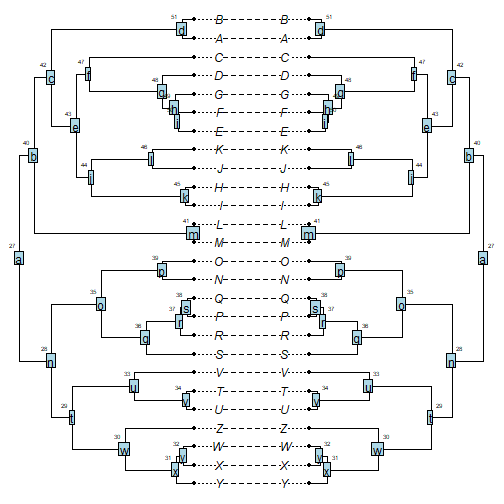So what was the problem with the edge labels in the user's inquiry? Well, basically, `edgelabels` (and `edgelabels.cophylo`, which just uses `edgelabels` internally) takes the labels in the order of the rows of `tree\$edge` in the plotted phylogeny

• not in the number order of the node indices, as in `nodelabels` and `tree\$node.label`. Thus, we have to match the two in order to visualize our node labels along each preceding edge. This might be done as follows:
``````obj<-cophylo(t1,t2,rotate=TRUE)
``````
``````## Rotating nodes to optimize matching...
## Done.
``````
``````plot(obj)
edgelabels.cophylo(obj\$trees[]\$node.label[2:obj\$trees[]\$Nnode],
edge=sapply(2:obj\$trees[]\$Nnode+Ntip(obj\$trees[]),
function(n,e) which(e==n),e=obj\$trees[]\$edge[,2]),
edgelabels.cophylo(obj\$trees[]\$node.label[2:obj\$trees[]\$Nnode],
edge=sapply(2:obj\$trees[]\$Nnode+Ntip(obj\$trees[]),
function(n,e) which(e==n),e=obj\$trees[]\$edge[,2]),
``````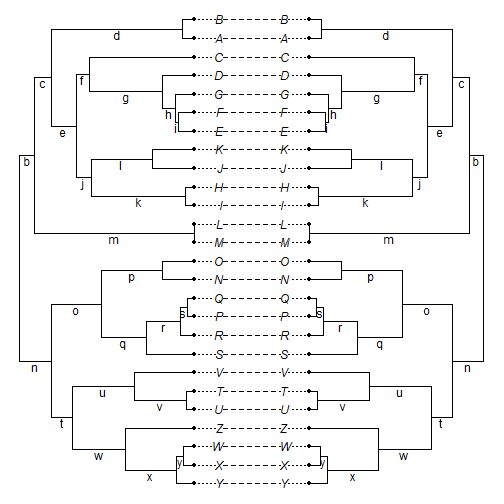Neat.

1.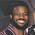Hello Liam,

Thank you for putting up this blog, it is very informative personally to me who is graduate student in bioinformatics who's just learning how to use R for phylogenetic studies.
My major challenge here is knowing when, where and how to use these functions (for example nodelabels, edgelabels, Nnode, untagle, and many more). Do you have any tips that you can share on how to use them appropriately and not mixing them up.
Cheers,
ID.

2.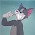Buy hublot fake watch, best breitling replica, breitling replica rolex watches , breitling replikas, u-boat italo fontana, replica cartier, hublot replica watches, fake watches breitling, replica montblanc, come here buy replica watches uk, rolex watches fake, watch replica breitling, replica tag heuer watches, omega watch copy, cheapest fake rolex, omega.

Note: due to the very large amount of spam, all comments are now automatically submitted for moderation.